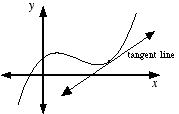index: click on a letter A B C D E F G H I J K L M N O P Q R S T U V W X Y Z A to Z index index: subject areas numbers & symbols sets, logic, proofs geometry algebra trigonometry advanced algebra & pre-calculus calculus advanced topics probability & statistics real world applications multimedia entrieswww.mathwords.com about mathwords website feedback

 Tangent Line A line that touches a curve at a point without crossing over. Formally, it is a line which intersects a differentiable curve at a point where the slope of the curve equals the slope of the line. Note: A line tangent to a circle is perpendicular to the radius to the point of tangency.